Courses

Current Electricity MCQ Level - 1

10 Questions MCQ Test Topic wise Tests for IIT JAM Physics | Current Electricity MCQ Level - 1

Description
Attempt Current Electricity MCQ Level - 1 | 10 questions in 30 minutes | Mock test for Physics preparation | Free important questions MCQ to study Topic wise Tests for IIT JAM Physics for Physics Exam | Download free PDF with solutions
QUESTION: 1

The electric cell does 5 Joule of work in carrying 10 C of charge around a close electric circuit. The electromotive force of the cell is :

Solution:

We know, E = W/Q where W = 5 joule, Q = 10 C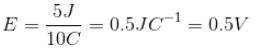QUESTION: 2

An energy source will supply a constant current into the load if its internal resistance is

Solution:

In case of an ideal battery, the internal resistance is zero.

QUESTION: 3

When current is passed through a wire heat is produced due to

Solution:

The correct answer is: collision of conduction electron with atoms.

QUESTION: 4

If a good conductor is connected between a point of an electric circuit and the ground.

Solution:

The correct answers are: the potential of the point is the same as that of the earth., current flows through the conductor only if one more point of the same circuit with some resistance between the two is connected to the ground

QUESTION: 5

When a wire of uniform cross-section a, length l and resistance is bent into a complete circle, resistance between any two of diametrically opposite points will be :

Solution:

The two half of the circle behave like two resistance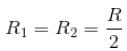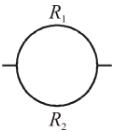Both resistance  R1  and  R2  are in parallel combination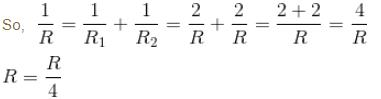QUESTION: 6

A heater coil is cut into two equals parts and only one part is now used in the heater. The heat generated will now be :

Solution:

We know, the relation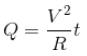When R  is halved, then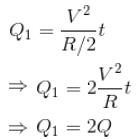QUESTION: 7

A steady current flows in a metallic conductor of non-uniform cross-section. The quantity/quantities constant along the length of the conductor is/are

Solution:

Drift speed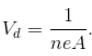Since A  is not constant therefore vd  is not constant.
The correct answer is: current only

QUESTION: 8

The electric resistance of a certain wire of iron is  R. If its length and radius are both doubled then

Solution:

The correct answer is: the resistance will be halved and the specific resistance will remain unchanged

QUESTION: 9

We are able to obtain fairly large current in a conductor because.

Solution:

The correct answer is: the number density of free electron is very high and this can compensate for the low values of the electron drift speed and the very small magnitude of the electron charge

QUESTION: 10

N  identical cells each of emf E  and internal resistance r, are joined in series to from a closed circuit. One cell A  is joined with reverse polarity. The potential difference across each cell except A  is

Solution:

Number of cells = n
EMF of each cell = ε
and Internal resistance of each cell =r
One cell is joined in reverse.
∴ Total EMF = n⋅ε − 2⋅ε
Current in the circuit, I = (n⋅ε − 2⋅ε)/n⋅r
Potential difference V = ε - I⋅r
ε -[ε⋅(n-2)/n⋅r]⋅r
= ε -[ε⋅(n-2)/n]
= [n⋅ε - n⋅ε + 2⋅ε]/n
= 2⋅ε/nUse Code STAYHOME200 and get INR 200 additional OFF Use Coupon Code

Track your progress, build streaks, highlight & save important lessons and more!

Similar Content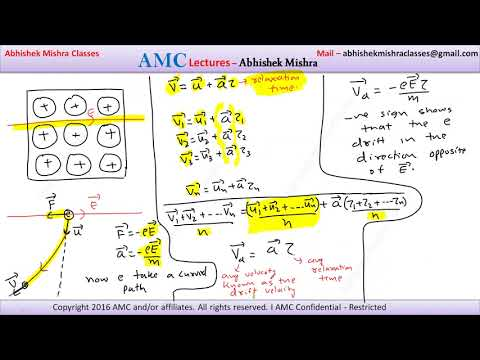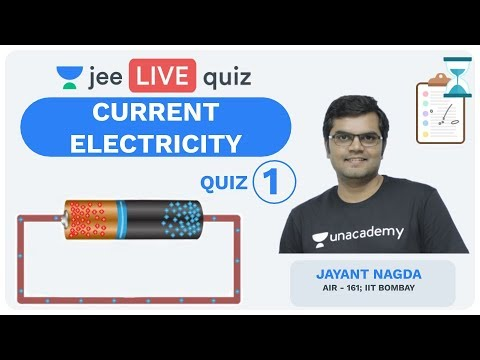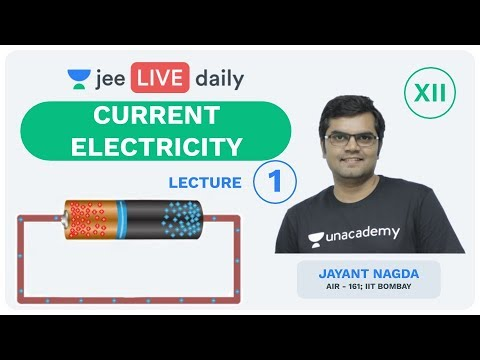Related tests